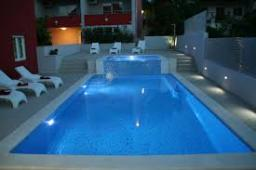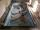# Rectangle pool

Determine dimensions of open pool with a square bottom with a capacity 32 m3 to have painted/bricked walls with least amount of material.

Correct result:

a =  4 m
c =  2 m

#### Solution:We would be pleased if you find an error in the word problem, spelling mistakes, or inaccuracies and send it to us. Thank you!Tips to related online calculators
Looking for help with calculating roots of a quadratic equation?
Do you have a linear equation or system of equations and looking for its solution? Or do you have quadratic equation?
Tip: Our volume units converter will help you with the conversion of volume units.

#### You need to know the following knowledge to solve this word math problem:

We encourage you to watch this tutorial video on this math problem:

## Next similar math problems:

• Alien shipThe alien ship has the shape of a sphere with a radius of r = 3000m, and its crew needs the ship to carry the collected research material in a cuboid box with a square base. Determine the length of the base and (and height h) so that the box has the large
• Body diagonalThe cuboid has a volume of 32 cm3. Its side surface area is double as one of the square bases. What is the length of the body diagonal?
• Uboid volumeCalculate the cuboid volume if the walls are 30cm², 35cm², 42cm²
• Cylindrical containerAn open-topped cylindrical container has a volume of V = 3140 cm3. Find the cylinder dimensions (radius of base r, height v) so that the least material is needed to form the container.
• Cuboid to cubeA cuboid with dimensions of 9 cm, 6 cm, and 4 cm has the same volume as a cube. Calculate the surface of this cube.
• Swimming poolThe pool shape of cuboid is 299 m3 full of water. Determine the dimensions of its bottom if water depth is 282 cm and one bottom dimension is 4.7 m greater than the second.
• Prism basesVolume perpendicular quadrilateral prism is 360 cm3. The edges of the base and height of the prism are in the ratio 5:4:2 Determine the area of the base and walls of the prism.
• Magnified cubeIf the lengths of the edges of the cube are extended by 5 cm, its volume will increase by 485 cm3. Determine the surface of both the original and the magnified cube.
• Secret treasureScouts have a tent in the shape of a regular quadrilateral pyramid with a side of the base 4 m and a height of 3 m. Determine the radius r (and height h) of the container so that they can hide the largest possible treasure.
• PoolThe prism-shaped pool is 2 m deep with a bottom of the isosceles trapezoid with base dimensions of 10 m and 18 m and arm legs 7 m long and 5.7 m long. During the spring cleaning, the bottom and walls of the pool must be painted. How many m2 of paint shoul
• Prism XThe prism with the edges of the lengths x cm, 2x cm and 3x cm has volume 20250 cm3. What is the area of surface of the prism?
• Pool coatingHow many pieces of tiles 25 cm × 15 cm need to coat the bottom and side walls of the pool with bottom dimensions 30 m × 5 m, if the pool can fit up to 271500 liters of water?
• PaintingTo paint the pool with dimensions: 2 meters depth, 3m x 4m we bought paint to 50 meters square. How many "paint" will be a waste?
• Cylinder - areaThe diameter of the cylinder is one-third the length of the height of the cylinder. Calculate the surface of cylinder if its volume is 2 m3.
• BallsThree metal balls with volumes V1=71 cm3 V2=78 cm3 and V3=64 cm3 melted into one ball. Determine it's surface area.
• Chemical parisonThe blown parison (with shape of a sphere) have a volume 1.5 liters. What is its surface?
• Cuboid and ratioFind the dimensions of a cuboid having a volume of 810 cm3 if the lengths of its edges coming from the same vertex are in ratio 2: 3: 5